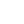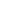# Methodology

The ISET Gender Portal features two Gender Equality Indexes: The Gender Equality Index for the South Caucasus (SCGE) and the Gender Equality Index for the Region in Transition (RTGEI). Both Indexes are modeled after the Gender Equality Index, developed by the European Institute for Gender Equality (EIGE) for the European Union.

The ISET Gender Equality Index for South Caucasus countries (SCGEI) combines 6 core domains: (1) work, (2) money, (3) knowledge, (4) time, (5) power, and (6) health. Each domain is comprised of sub-domains (11 sub-domains in total), which include indicators (19 indicators in total) from international and national (country reports) sources. Gender Equality Index covers three South Caucasus countries: Georgia, Armenia, and Azerbaijan, and compares their outcomes to the 9 benchmark countries: Bulgaria, Czech Republic, Estonia, Hungary, Latvia, Lithuania, Poland, Romania, and Slovenia. These countries were chosen as a benchmark/comparator set for the South Caucasus because they share a common legacy of transition with the three South Caucasus countries and represent the transition economies which have since joined the European Union.

The ISET Gender Equality Index for Region in Transition (RTGEI) covers 12 countries in Europe and Central Asia which are either in the process of transition or have already transitioned from the soviet economic and social model (Armenia, Azerbaijan, Belarus, Estonia, Georgia, Kazakhstan, Kyrgyzstan, Latvia, Lithuania, Moldova, Russia, Ukraine), with two EU countries included as comparators: Sweden (appears at the top of EU gender equality indicators) and Greece (the country that appears at the bottom of EU gender equality rankings). RTGEI is also comprised of 6 core domains: (1) work, (2) money, (3) knowledge, (4) time, (5) power, and (6) health; however, the indicators used in the domains differ from the ones used in SCGEI, which is mostly due to data availability issues for a large number of countries in the RTGEI set.

The process of calculating GE Index includes the following steps: (1) selection and processing of indicators, (2) calculating gender gaps, (3) calculating the correction coefficient, (4) calculating he gender gap metric, and (5) calculating the index.

##### 1. Selection and processing of indicators

The SCGEI and RTGEI Indices include 19 and 16 indicators respectively (each for women and men), ensuring that variables measure gender equality in a homogenous way (having the same interpretation). The missing data is imputed by the simple method of taking the close value of the variable.

2. Calculating gender gaps

The gender gap is calculated by the following formula: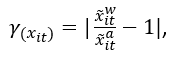Where the calculation is carried out for the variable X for the i-th country in the period t in order to obtain the percentage that women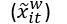represent over the country average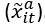. The gender gap takes values between 0 and 1, where 0 stands for complete gender equality and 1 – for full gender inequality.

3. Calculating the correction coefficient

The correction coefficient is calculated by the following formula: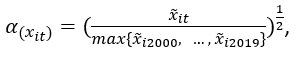The correcting coefficient makes it possible to take into account the country context by comparing the levels achieved in all countries for each indicator. The correcting coefficient takes values between 0 and 1 and for each indicator penalizes countries with low overall achievement.

4. Calculating he gender gap metric

The gender gap metric is calculated by the following formula: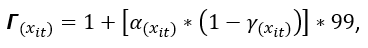The original variables disaggregated by sex are replaced by their transformation through a metric, which is dimensionless. It varies from 1 to 100 and shows the distance of each variable from the equality point, set at 100.

5. Calculating the index

Indicators are aggregated using the arithmetic mean and weighted equally. Sub-domains and domains are aggregated using the geometric mean. Moreover, sub-domains are weighted equally, while domains are weighted using mean experts’ judgment, as reported by EIGE (the table of expert-determined weights is provided below).

 Work Money Knowledge Time Power Health 0.19 0.15 0.22 0.15 0.19 0.10

Note that more details on the methodology for both indexes, indicators and data sources are provided in the ppt files below.

South Caucasus - Gender Index Methodology

Region in Transition - Gender Index Methodology